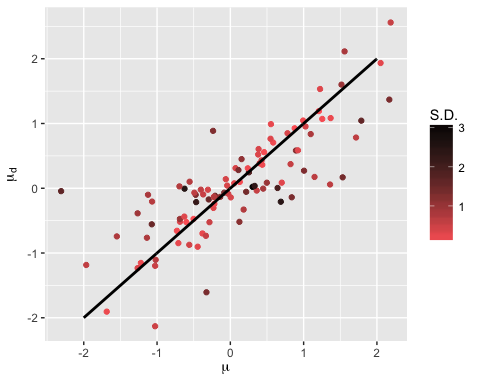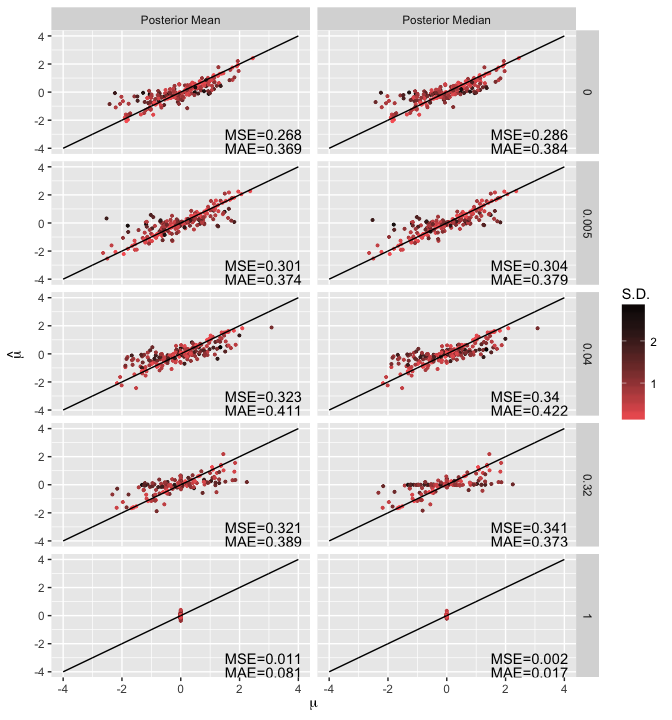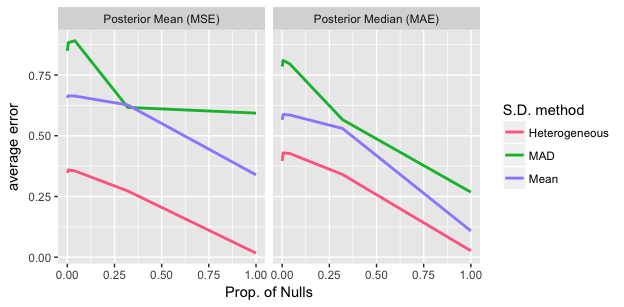# An illustration of EbayesThresh with heterogeneous variance

#### 2017-06-07

This vignette demonstrates EbayesThresh with heterogeneous variance. This is an extension of the original EbayesThresh developed by Johnstone and Silverman.

First, load the necessary packages into the R environment.

library(EbayesThresh)
library(ggplot2)
library(dplyr)

We start with a simple example of data sequence simulated with Gaussian noise and heterogeneous standard deviations.

set.seed(123)
mu = rnorm(100)                 # True effects
sd = sqrt(rchisq(100, df = 1))  # Sampling standard deviation
x  = mu + rnorm(100, sd = sd)   # Data sequence

Next, we plot the estimated effects using posterior median ($$\mu_d$$) versus the true effects ($$\mu$$), colored according to the sampling standard deviation, with high standard deviation being black and low standard deviation being red. Posterior medians of observations with larger standard deviation are closer to zero. Intuitively, larger standard deviation implies more uncertainty and less information and, thus, posterior estimates will be closer to the prior.

# Using Laplace prior and posterior median as estimator, with
# constraints on thresholds.
x.est = ebayesthresh(x,prior = "laplace",a = NA,sdev = sd,verbose = TRUE,
threshrule = "median")
ggplot(data = data.frame(x = mu, y = x.est$muhat, s = sd)) + geom_point(aes(x=x, y=y, col=s)) + geom_line(data = data.frame(x = seq(-2, 2, 0.01)), aes(x = x, y = x),size = 1) + scale_colour_gradient(name = "S.D.", low = "#FF6666", high = "black") + xlab(expression(mu)) + ylab(expression(mu[d]))Now we demonstrate the performance of EbayesThresh with heterogeneous variance using posterior mean/median with different proportions of null effects (with constraints on threshold). Consider a data sequence of 2,000 observations with $$m$$ null effects and $$2,000 - m$$ effects drawn from $$N(0, 1)$$, in which $$m = 0, 10, 80, 640$$, and $$2000$$. Each true effect $$\mu_i$$ is observed with noise drawn from a normal distribution $$N(0, s_i^2)$$, with $$s_i^2 \sim \chi^2_1$$. ebt_est = function(data, threshrule="median", universalthresh=TRUE, stabadjustment=FALSE){ mu_hat = c() for(i in 1:max(data$group)){
x = data[data$group==i, "x"] s = data[data$group==i, "sd"]
x.est = ebayesthresh(x,"laplace",a=NA,sdev=s,threshrule=threshrule,
verbose=TRUE,universalthresh=universalthresh,
mu_hat = c(mu_hat, x.est$muhat) } data$mu_hat = mu_hat
return(data)
}

# Datasets with different proportions of null effects.
nobs   = 2000
nzeros = c(0, 10, 80, 640, 2000)
weight = (nobs - nzeros)/nobs
mu_vec = c()
sd_vec = c()
x_vec  = c()
group  = c()
group_label = c()
set.seed(123)
for(i in 1:length(nzeros)){
s           = sqrt(rchisq(nobs, 1))
mu          = c(rep(0, nzeros[i]), rnorm(nobs - nzeros[i]))
x           = mu + rnorm(nobs,0,s)
sd_vec      = c(sd_vec, s)
mu_vec      = c(mu_vec, mu)
x_vec       = c(x_vec, x)
group       = c(group, rep(i, nobs))
group_label = c(group_label, rep(nzeros[i]/nobs, nobs))
}
data = data.frame(mu=mu_vec, sd=sd_vec, x=x_vec, group, group_label)

The estimation results of $$m = 0, 10, 80, 640$$, and $$2000$$ (corresponding to proportion $$0, 0.005, 0.04, 0.32$$ and $$1$$) are plotted every 10 data points ( $$10^{th}, 20^{th} \cdots$$ of the observations ) versus the true effects. Mean squared error (MSE) and mean absolute error (MAE) are shown in each panel. We can see that posterior estimates of observations with larger standard deviation are closer to zero in almost all the panels.

# Estimate the true effects with posterior mean/median.
data.med_est         = ebt_est(data, "median")
data.med_est$method = "Posterior Median" data.mean_est = ebt_est(data, "mean") data.mean_est$method = "Posterior Mean"
data.est             = rbind(data.med_est, data.mean_est)

# Calculate MSE/MAE for each dataset and each posterior estimator.
data.plot=data.est[row(data.est)[, 1] %% 10==0, ]
data.plot_sum =
as.data.frame(data.plot %>% group_by(group_label, method) %>%
summarise(mse=mean((mu_hat-mu)^2),mae=mean(abs(mu_hat-mu))))
data.plot_sum$label1 = paste("MSE=", round(data.plot_sum$mse, 3), sep="")
data.plot_sum$label2 = paste("MAE=", round(data.plot_sum$mae, 3), sep="")

ggplot(data.plot) +
geom_point(aes(x=mu, y=mu_hat, col=sd), size=0.7) +
facet_grid(group_label~method) +
geom_line(data=data.frame(x=seq(-4, 4, 0.01)), aes(x=x, y=x)) +
geom_text(x = 1.5, y = -3, aes(label=label1), data=data.plot_sum, hjust=0) +
geom_text(x = 1.5, y = -4, aes(label=label2), data=data.plot_sum, hjust=0) +
xlab(expression(mu)) +
ylab(expression(hat(mu)))Now, we compare MSE and MAE using posterior mean and median respectively over different proportions of null effects with different measures of standard deviation. Three different standard deviations are passed to the model: i) homogeneous standard deviation measured by median absolute deviation (MAD) of the observations, ii) homogeneous standard deviation measured by mean of the true standard deviations, and iii) the true heterogeneous standard deviations. For each proportion of null effects and each standard deviation, we calculate MSE and MAE 10 times and then take the average.

ebt_error = function(data, threshrule="median", nsim = 10){
mse_mat = matrix(0, nrow=3, ncol=length(nzeros))
mae_mat = matrix(0, nrow=3, ncol=length(nzeros))
for(i in 1:length(nzeros)){
mse_vec = 0; mae_vec = 0
for(n in 1:nsim){
s = sqrt(rchisq(nobs, 1))
mu = data[data$group==i, "mu"] x = mu + rnorm(nobs,0,s) x.est = ebayesthresh(x,"laplace",a=NA,sdev=s,threshrule=threshrule, verbose=TRUE) x.est_mad = ebayesthresh(x,"laplace",a=NA,sdev=NA,threshrule=threshrule, verbose=TRUE) x.est_mean = ebayesthresh(x,"laplace",a=NA,sdev=mean(s), threshrule=threshrule,verbose=TRUE) mse_vec = mse_vec + c(mean((mu - x.est$muhat)^2),
mean((mu - x.est_mad$muhat)^2), mean((mu - x.est_mean$muhat)^2))
mae_vec = mae_vec + c(mean(abs(mu - x.est$muhat)), mean(abs(mu - x.est_mad$muhat)),
mean(abs(mu - x.est_mean$muhat))) } mse_vec = mse_vec/nsim mae_vec = mae_vec/nsim mse_mat[, i] = mse_vec mae_mat[, i] = mae_vec } return(list(mse_mat=mse_mat, mae_mat=mae_mat)) } # Estimation of MSE/MAE for posterior mean/median with different non-null # weights. set.seed(123) error_mat.med_est = ebt_error(data, "median") error_mat.med_mae = error_mat.med_est$mae_mat
error_mat.mean_est = ebt_error(data, "mean")
error_mat.mean_mse = error_mat.mean_est\$mse_mat

We find that easing the initial restriction of homogeneous standard deviation can greatly improve the performance of empirical bayesian thresholding method in terms of MSE and MAE.

data.error_plot =
data.frame(mse=c(t(error_mat.med_mae),t(error_mat.mean_mse)),
nzeros=rep(nzeros/nobs, 3*2),
xlab("Prop. of Nulls") + ylab("average error")